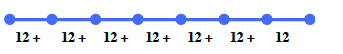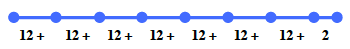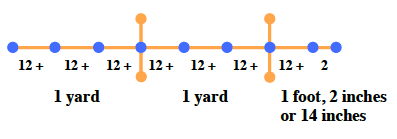### Home > MC1 > Chapter 2 > Lesson 2.1.2 > Problem2-19

2-19.

Terrance knows that $12$ inches is the same length as $1$ foot and that $1$ yard is the same length as $3$ feet.

1. Terrance measures a board and finds that it is $86$ inches long. How can he use feet and/or yards to help express this length? Is there more than one way he can do this? Use a diagram to help explain your answers.

Begin by counting by $12$ inches and see how close you can get to $86$ inches, without going over.

Next, add two more inches to make the length equal to $86$ inches.

Count the number of yards in the diagram. Remember that one yard is equal to three feet, or $36$ inches.

$86$ inches can be expressed as $7$ feet, $2$ inches;
$2$ yards, $1$ foot, $2$ inches; or $2$ yards, $14$ inches.
The diagram above helps explain this answer.1. Terrance's brother is $2$ yards tall. How else can his height be expressed? Use a diagram to help support your answers.

Try drawing a diagram like the one for part (a). This will help you
visualize how many feet (and inches) are in $2$ yards.